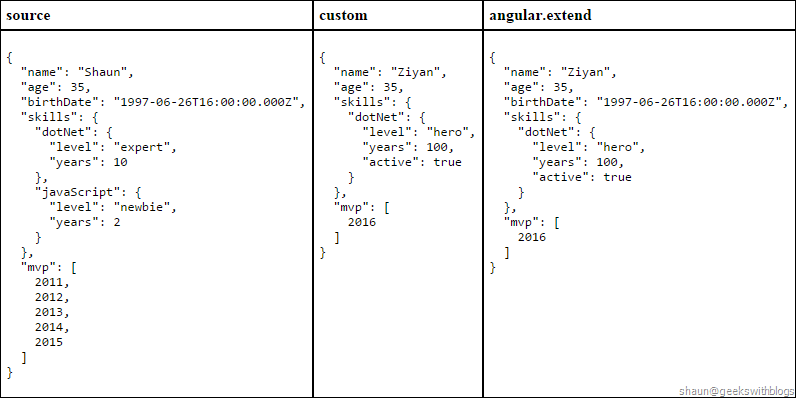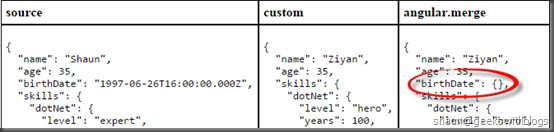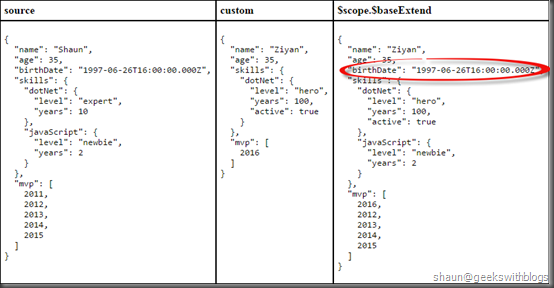# 【译】在时间属性上慎用Angular.js1.4的“angular.merge”函数 0个回复专栏@ Javascript

`````` \$scope.source = {
name: 'Shaun',
age: 35,
birthDate: new Date(1997, 5, 27),
skills: {
dotNet: {
level: 'expert',
years: 10
},
javaScript: {
level: 'newbie',
years: 2
}
},
mvp: [
2011,
2012,
2013,
2014,
2015
]
};

\$scope.custom = {
name: 'Ziyan',
age: 35,
skills: {
dotNet: {
level: 'hero',
years: 100,
active: true
},
},
mvp: [
2016
]
};

\$scope.extend = angular.extend({}, \$scope.source, \$scope.custom);
````````````\$scope.merge = angular.merge({}, \$scope.source, \$scope.custom);
````````````   function baseExtend(dst, objs, deep) {
var h = dst.\$\$hashKey;

for (var i = 0, ii = objs.length; i < ii; ++i) {
var obj = objs[i];
if (!isObject(obj) && !isFunction(obj)) continue;
var keys = Object.keys(obj);
for (var j = 0, jj = keys.length; j < jj; j++) {
var key = keys[j];
var src = obj[key];

if (deep && isObject(src)) {
if (!isObject(dst[key])) dst[key] = isArray(src) ? [] : {};
baseExtend(dst[key], [src], true);
} else {
dst[key] = src;
}
}
}

setHashKey(dst, h);
return dst;
}
``````

`````` \$scope.\$baseExtend = function (dst, objs, deep) {
for (var i = 0, ii = objs.length; i < ii; ++i) {
var obj = objs[i];
if (!angular.isObject(obj) && !angular.isFunction(obj)) {
console.log('[' + obj + '] = (skip)');
continue;
}
var keys = Object.keys(obj);
for (var j = 0, jj = keys.length; j < jj; j++) {
var key = keys[j];
var src = obj[key];
// perform deep copy if
// 1. spcified by user
// 2. source property is an object
// 3. source property is NOT a date
if (deep && angular.isObject(src) && !angular.isDate(src)) {
if (!angular.isObject(dst[key])) {
console.log('[' + key + '] = (Try copy an object to an non-object, create an empty for deep copy.)');
dst[key] = angular.isArray(src) ? [] : {};
}
\$scope.\$baseExtend(dst[key], [src], true);
}
else {
dst[key] = src;

console.log('[' + key + '] = ' + src);
}
}
}
return dst;
};

\$scope.sample = \$scope.\$baseExtend({}, [\$scope.source, \$scope.custom], true);
``````